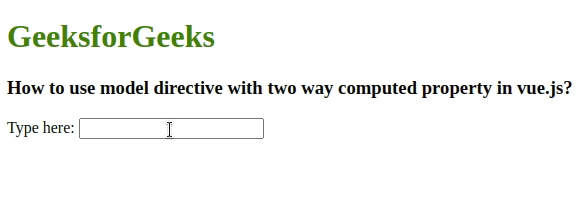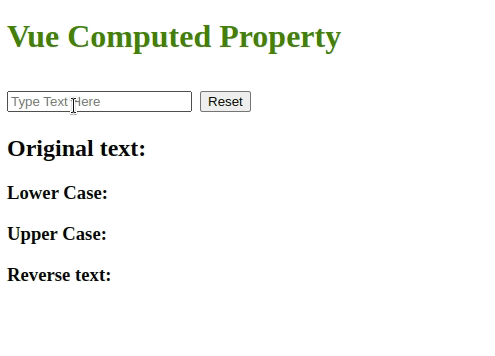GeeksforGeeks App
Open AppBrowser
Continue

# How to use model directive with two way computed property in vue.js ?

Vue.js is an open-source Model–View–View Model front-end JavaScript framework for building user interfaces and single-page applications. Data binding is one of the most important features in web applications. Vue.js provides two-way data binding that helps DOM to update automatically when the data Model is changed. Vue.js uses a v-model directive for two-way data binding so no need to write complex code for two-way data binding, unlike other frameworks. In this article, we will see how to use a v-model directive with two-way computed property in vue.js.

The v-model is used for two-way data flow and reactive data binding. it uses internally inside the HTML attributes which can bind along with data enclosed by double curly braces

Syntax: The input field uses a v-model directive, whenever we enter a character in the input field, it will render automatically inside <p> tag.

```<input v-model="name" />
<h1>{{name}}</h1>```

Approach 1: Here, the text that is entered in an input Text field, the Vue() constructor initiates the data objects for {{name}} as an empty string inside the <script> element. In the input field whenever we enter a character it will render the same in {{name}} expression by the v-model directive.

Example 1: In the below example, we are creating two-way binding data using the v-model directive.

## HTML

 ```<``html``>`` ` `<``head``>``    ``<``title``>``        ``Two-way computed property in VueJS``    ````    ``<``script` `src``=``"https://cdn.jsdelivr.net/npm/vue@2.7.14/dist/vue.js"``>``    `````` ` `<``body``>``    ``<``h1` `style``=``"color: green;"``>``        ``GeeksforGeeks``    ````    ``<``h3``>``        ``How to use model directive with two ``        ``way computed property in vue.js?``    ````    ``<``div` `id``=``'firstApp'``> Type here:``        ``<``input` `v-model``=``"name"` `/>``        ``<``h1``>{{name}}``        ``<``div``>``            ``<``script``>``                ``const firstApp = new Vue({``                    ``el: '#firstApp',``                    ``data: {``                        ``name: ''``                    ``}``                ``});``           ````          ````      `````` ` ``

Output:In case we need to convert the name into upper case, it actually coded like the below format inside the DOM elements.

`<p>{{name.toUpperCase()}}</p>`

or, if you initiate the upper text as data objects inside Vue() constructor.

```data: {
name: '',
upperText: name.toUpperCase()
}```

But these approaches will throw an error that “ReferenceError: name is not defined”. To overcome this error, we are using computed properties when javascript objects need logic in the data model.

The Computed property is reactive data to calculate and display values based on the data model objects. It enables the creation of a data property that can be used to modify, manipulate and display data within your components. It is one of the components in the Vue() constructor.

Syntax:

```data: {
name: ''
},
computed: {
}```

Vue() constructor initiates Vue components for interacting with DOM. The components have special cases that consist el, data, methods, and computed. Vue.js detect the Vue() constructor and updates the data model through Vue Components.

• el: it is used for binding HTML elements
• data: it is used for binding the javascript objects
• methods { }: it is used to bind the javascript functions
• computed { }: it is used to bind the properties which are computed by the data model
```<script>
const firstApp = new Vue({
el:'#firstApp',
data: {
name: ''
},
computed: {},
methods: {}
});
</script>```

Now, we are creating a computed property for v-model two-way binding data in the Vue component. Add the upper case text logic inside computed:{ } block as computed property is shown below.

```computed: {
upperText: function() {
return this.name.toUpperCase();
}
}```

Bind the computed property as a string value inside the double curly braces expression. whenever we enter any text in an input field, the v-model will render the name with two-way binding and the computed property will convert the name into upper case.

```<input v-model="name" />
<p>{{upperText}}</p>```

Instead of a computed property, we can define the same function as a method, but it is not reactive data binding. if we use a method need to trigger it for each evaluation, but computed properties are cached based on their reactive dependencies. so it re-evaluates the data model when some of its reactive dependencies have changed.

Approach 2: Here, we are creating three computed properties for the input text which is bonded with the v-model directive. First, create an input text box with the v-model directive and a button in the HTML file. Add Vue Components in the javascript file. Create a Vue () constructor and it initiates Vue Components for interacting with DOM. first computed property converts the input text into lower case, the second one will convert into upper case and the third one will convert into palindrome text(reverse order). Additionally, add a button for resetting everything and bind the button click event with Vue component methods.

Example 2: This example illustrates the implementation of two-way data binding using computed properties.

• index.html

## HTML

 ```<``html``>`` ` `<``head``>``    ``<``title``>``          ``Two-way computed property in Vue.js``      ````    ``<``script` `src``=``"https://cdn.jsdelivr.net/npm/vue@2.7.14/dist/vue.js"``>``    ````    ``<``style``>``        ``span, h1 {``            ``color: green;``        ``}``    `````` ` `<``body``>``    ``<``div` `id``=``'firstApp'``>``        ``<``h1``>{{header}}``        ``<``input` `v-model``=``"sampleText"` `               ``placeholder``=``'Type Text Here'` `/>``        ``<``button` `v-on:click``=``"firstBtn"``>``            ``Reset``        ````        ``<``h2``>Original text:``            ``<``span``>{{sampleText}}``        ````        ``<``h3``> Lower Case:``            ``<``span``>{{lowerText}}``        ````        ``<``h3``> Upper Case:``            ``<``span``>{{upperText}}``        ````        ``<``h3``>Reverse text:``            ``<``span``>{{reverseText}}``        ````    ````    ``<``script` `src``=``'app.js'``>```` ` ``

• app.js

## Javascript

 `const firstApp = ``new` `Vue({``    ``el: ``'#firstApp'``,``    ``data: {``        ``sampleText: ``''``,``        ``header: ``'Vue Computed Property'``    ``},``    ``// computed properties``    ``computed: {``        ``lowerText: ``function` `() {``            ``return` `this``.sampleText.toLowerCase();``        ``},``        ``upperText: ``function` `() {``            ``return` `this``.sampleText.toUpperCase();``        ``},``        ``reverseText: ``function` `() {``            ``return` `this``.sampleText.split(``''``).reverse().join(``''``);``        ``}``    ``},``    ``// This method will clear the data ``    ``// field using button event click``    ``methods: {``        ``firstBtn: ``function` `() {``            ``this``.sampleText = ``''``;``        ``}``    ``}``});`

Output:My Personal Notes arrow_drop_up
Related Tutorials